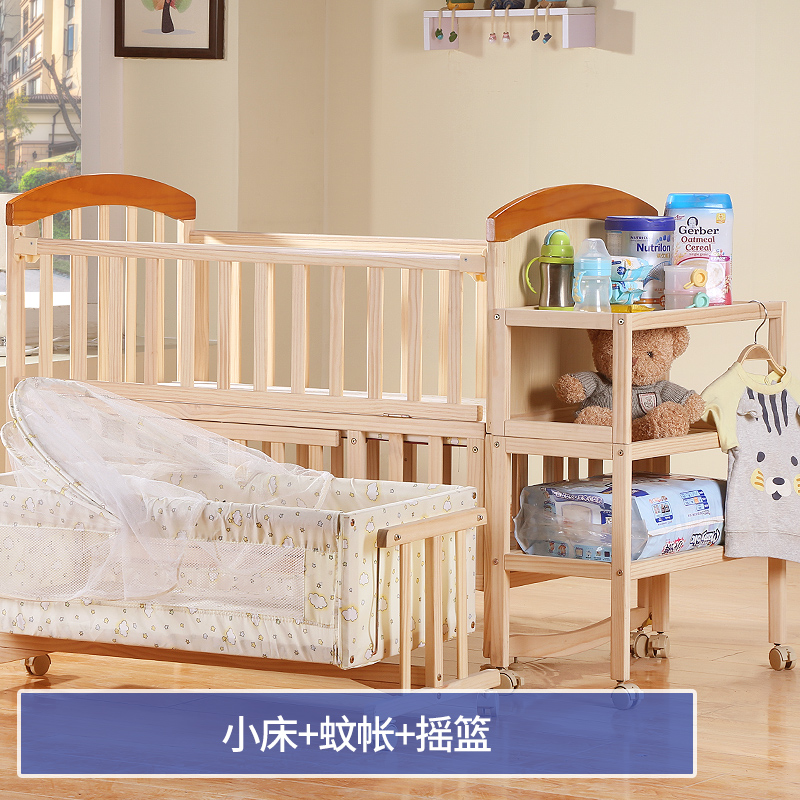B a b y : B a b y b e d s o l i d w o o d c r a d l e p a i n t l e s s c h i l d r e n s t i t c h e d b e d m u l t i - p u r p o s e b a b y b b n e w b o r n

Updated Wednesday 09-Oct-2019B a b y : B a b y b e d s o l i d w o o d c r a d l e p a i n t l e s s c h i l d r e n s t i t c h e d b e d m u l t i - p u r p o s e b a b y b b n e w b o r n 0

THB 4,695.23

More details at ezbuy.co.th